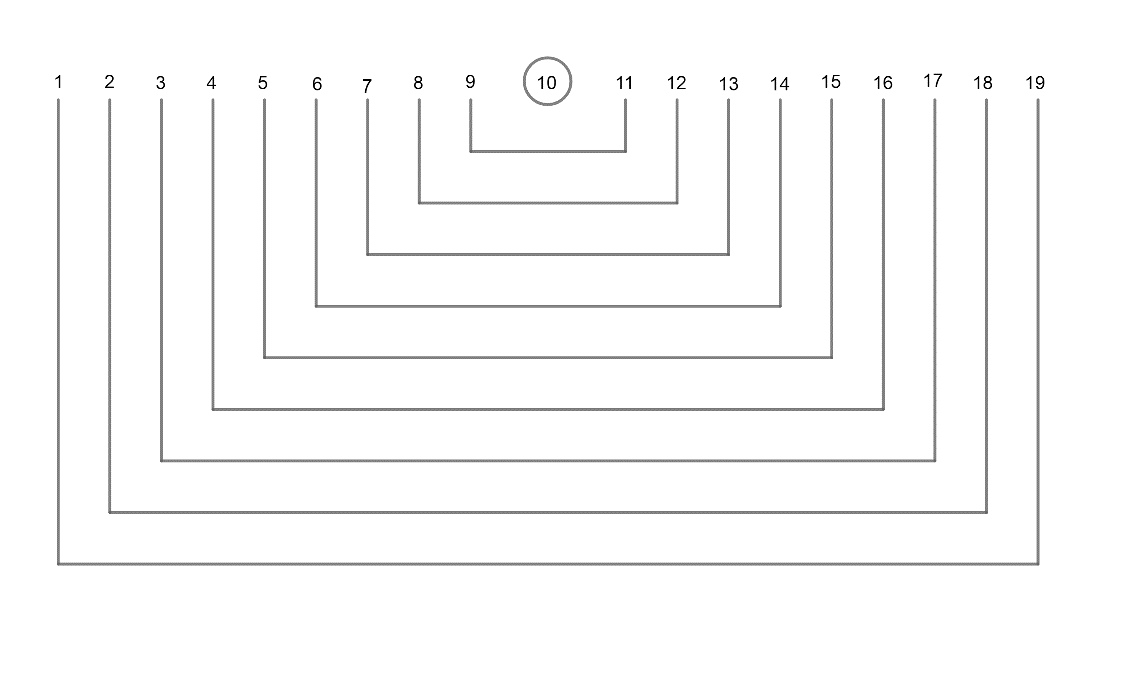Courses
Courses for Kids
Free study material
Offline Centres
MoreLast updated date: 27th Nov 2023
Total views: 380.7k
Views today: 7.80k

# If x, y, z are three natural numbers in A.P. such that $x+y+z=30$ then the possible number of ordered triplet (x, y, z) isA. 18B. 19C. 20D. 21Verified
380.7k+ views
Hint: Take the common difference as ‘d’. Substitute the values of x, y and z in the equation $x+y+z=30$. From the simplified equation, find the AP and substitute different values of d, which gives the total number of possibilities of ordered triplet.

It is said that x, y and z represent 3 natural numbers in AP.
AP represents Arithmetic progression. It is a sequence of numbers such that the difference between the consecutive terms is constant.
Difference here means the second minus the first term.
Let us consider ‘d’ as the common difference of the Arithmetic progression and ‘d’ is a natural number.
Here ‘x’ is the first term of AP.
y is the 2nd term of AP and ‘z’ the 3rd term.
Now, we need to find the difference between consecutive terms.
So, 2nd term – 1st term = d
$\Rightarrow y-x=d$
Rearrange the terms $x=y-d$.
Similarly, 3rd term – 2nd term = d.
$z-y=d\Rightarrow z=y+d$
Now, substitute the value of x and z in the equation $x+y+z=30$.
\begin{align} & \Rightarrow \left( y-d \right)+y+\left( y+d \right)=30 \\ & \Rightarrow y-d+y+y+d=30 \\ & \Rightarrow y+y+y=30 \\ & \Rightarrow 3y=30 \\ & \therefore y=\dfrac{30}{3}=10 \\ & \therefore x=y-d=10-d \\ & z=y+d=10+d \\ \end{align}
$\therefore$ The AP of x, y, z is now equal to (10 – d), 10, (10 + d).
Now, let’s consider d =1, then AP becomes 9, 10, 11.
Similarly,
d = 2, AP = 8, 10, 12
d = 3, AP = 7, 10, 13
d = 4, AP = 6, 10, 14
d = 5, AP = 5, 10, 15
d = 6, AP = 4, 10, 16
d = 7, AP = 3, 10, 17
d = 8, AP = 2, 10, 18
d = 9, AP = 1, 10, 19
So these combinations of ordered triplets are possible.
i.e. now there are 9 possibilities which are the same for (19, 1), (18, 2)…..
$\therefore$ Number of possibilities $=9\times 2=18$ possibilities
Hence option A is the correct answer.

Note: The possibilities available can be represented as